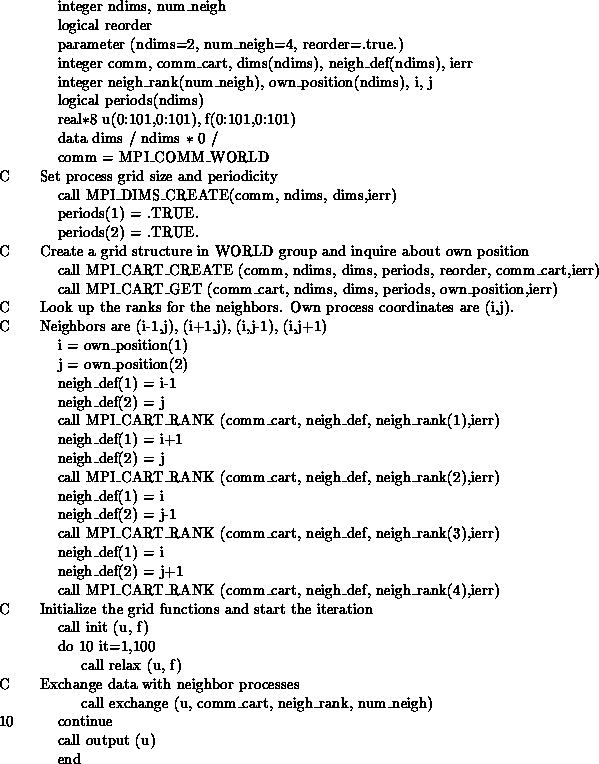# 6.6. An Application ExampleUp: Process Topologies Next: MPI Environmental Management Previous: Low-level topology functions

Example

The example in figure An Application Example shows how the grid definition and inquiry functions can be used in an application program. A partial differential equation, for instance the Poisson equation, is to be solved on a rectangular domain. First, the processes organize themselves in a two-dimensional structure. Each process then inquires about the ranks of its neighbors in the four directions (up, down, right, left). The numerical problem is solved by an iterative method, the details of which are hidden in the subroutine relax.

In each relaxation step each process computes new values for the solution grid function at all points owned by the process. Then the values at inter-process boundaries have to be exchanged with neighboring processes. For example, the exchange subroutine might contain a call like MPI_SEND(...,neigh_rank(1),...) to send updated values to the left-hand neighbor (i-1,j).Set-up of process structure for two-dimensional parallel Poisson solver.Up: Process Topologies Next: MPI Environmental Management Previous: Low-level topology functions